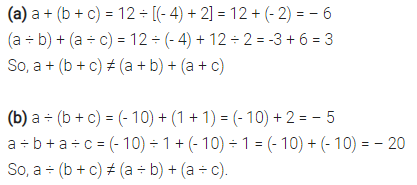# Verify that a + (b + c) ≠ (a + b) + (a ÷ c)

Verify that
a + (b + c) ≠ (a + b) + (a ÷ c)
for each of the following values of a, b and c.
(a) a = 12, b = – 4, c = 2
(b) a = (- 10), b = 1, c = l.